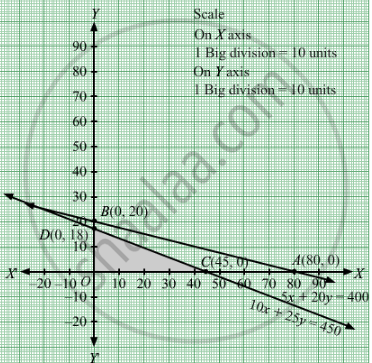# A Furniture Manufacturing Company Plans to Make Two Products : Chairs and Tables. from Its Available Resources Which Consists of 400 Square Feet to Teak Wood and 450 Man Hours. - Mathematics

Sum

A furniture manufacturing company plans to make two products : chairs and tables. From its available resources which consists of 400 square feet to teak wood and 450 man hours. It is known that to make a chair requires 5 square feet of wood and 10 man-hours and yields a profit of Rs 45, while each table uses 20 square feet of wood and 25 man-hours and yields a profit of Rs 80. How many items of each product should be produced by the company so that the profit is maximum?

#### Solution

Let  units of chairs and y units of tables were produced Therefore  $x, y \geq 0$

The given information can be tabulated as follows:

 Wood(square feet) Man hours Chairs(x) 5 10 Tables(y) 20 25 Availability 400 450

Therefore, the constraints are

$5x + 20y \leq 400$
$10x + 25y \leq 450$
It is known that to make a chair requires 5 square feet of wood and 10 man-hours and yields a profit of Rs 45, while each table uses 20 square feet of wood and 25 man-hours and yields a profit of Rs 80.
Therefore, profit gained to make x chairs and y tables is Rs 45x and Rs 80y respectively.
Total profit = Z = $45x + 80y$

which is to be maximised.

Thus, the mathematical formulat​ion of the given linear programmimg problem is

Max Z =  $45x + 80y$

subject to

$5x + 20y \leq 400$
$10x + 25y \leq 450$

$x, y \geq 0$

First we will convert inequations into equations as follows:
5x + 20y = 400, 10x + 25y = 450, x = 0 and y = 0
Region represented by 5x + 20y ≤ 400:
The line 5x + 20y = 400 meets the coordinate axes at

$A\left( 80, 0 \right)$ and  $B\left( 0, 20 \right)$respectively. By joining these points we obtain the line
5x + 20y = 400 . Clearly (0,0) satisfies the 5x + 20y = 400 . So, the region which contains the origin represents the solution set of the inequation 5x + 20y ≤ 400.

Region represented by 10x + 25y ≤ 450:
The line 10x + 25y = 450 meets the coordinate axes at $C\left( 45, 0 \right)$ and $D\left( 0, 18 \right)$ respectively. By joining these points we obtain the line
10x + 25y = 450. Clearly (0,0) satisfies the inequation 10x + 25y ≤ 450. So,the region which contains the origin represents the solution set of the inequation 10x + 25y ≤ 450.
Region represented by x ≥ 0 and y ≥ 0:
Since, every point in the first quadrant satisfies these inequations. So, the first quadrant is the region represented by the inequations x ≥ 0, and ≥ 0.
The feasible region determined by the system of constraints 5x + 20y ≤ 400, 10x + 25y ≤ 450, x ≥ 0, and y ≥ 0 are as follows.The corner points are A(0, 18), B(45, 0)

The values of Z at these corner points are as follows

 Corner point Z= 45x + 80y A 1440 B 2025

The maximum value of Z is 2025 which is attained at $\left( 45, 0 \right)$ .

Thus, the maximum profit  is of Rs 2025 obtained when 45 units of chairs and no units of tables are produced

Concept: Graphical Method of Solving Linear Programming Problems
Is there an error in this question or solution?

#### APPEARS IN

RD Sharma Class 12 Maths
Chapter 30 Linear programming
Exercise 30.4 | Q 11 | Page 51